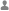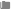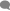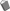## 记两题笔试题Veris分类：PHP0条评论已被围观 2088
```<?php
/**
*  1、我们的程序运行过程中每分钟会采集一个整数的数据指标。
* 	持续采集n分钟就得到一个有n个元素的整数数组a[n]。
* 	现在我们需要一个简单的算法，检测采集到的数据指标中，是否有异常。
* 	异常的检测标准是：如果在连续m分钟内的指标的平均值大于w，则说明有异常。
* 	输入：数组a[n], 正整数m, 整数w
* 	返回：有异常返回 1，没有异常返回 0
* 	例如：对于a={1, 5, 1, 3, 2}, m=2, w=2, 返回:1
* 	附加说明：不同的实现方式执行效率不一样，如果能找到一个很高效的算法就更好了。
*/

/**
* 检测函数.
*
* @param \$a     array 待测数组
* @param \$start int   检测时间起点
* @param \$m     int   检测时间范围
* @param \$w     int   平均指标标准
*
* @return int 状态
*/
function check(\$a, \$start, \$m, \$w)
{
\$n = count(\$a);
\$m > \$n && \$m = \$n;
\$arr = array_slice(\$a, \$start-1, \$m);
\$s = array_sum(\$arr);
if (\$s / \$m > \$w) {
return 1;
} else {
return 0;
}
}

/************** 测试 **************/
for (\$i=0; \$i < 1000; \$i++) {
\$a[]=rand(1,4);
}
\$aLenth = count(\$a);
\$m=5;
\$w=3;
echo '检测时间范围：'.\$m.'分钟，检测指标：'.\$w.'<br><hr>';
for (\$i=1; \$i <= \$aLenth; \$i++) {
\$arr=array_slice(\$a, \$i-1, \$m);
\$n=count(\$arr);
\$avg=array_sum(\$arr)/\$n;
echo '时间：' . \$i . '~' . (\$i+\$m-1) . '，数据：{' . implode(',', \$arr) . '}，平均值：'. \$avg;
if(check(\$a, \$i, \$m, \$w)==1){
echo '<font color="red">【异常】</font>';
}
echo '<br>';
}```

```<?php
/**
*  2、我们的程序运行过程中用到了一个数组a，数组元素是一个Map/Dictionary。
* 	数组元素的“键”和“值”都是字符串类型。在不同的语言中，对应的类型是：
* 	PHP的array, Java的HashMap, C++的std::map, Objective-C的NSDictionary, Swift的Dictionary,  * Python的dict, JavaScript的object, 等等
* 	示例：
* 	a["key1"]="value1"
* 	a["key2"]="value2"
* 	a["keyA"]="valueA"
* 	...
* 	为了方便保存和加载，我们使用了一个基于文本的存储结构，数组元素每行一个：
* 	text="key1=value1;key2=value2\nkeyA=valueA\n...".
*
* 	要求：请实现一个“保存”函数、一个“加载”函数。
* 	text=store(a);
* 	这两个函数分别用于把数组保存到一个文本字符串中、把文本字符串中的内容读取为数组。
* 	必须自己手写代码实现保存/加载逻辑，严格按照上述的“每行一个、key=value”的格式保存。
*
* 	附加说明：基于上述格式，如果value中有特殊字符，比如有换行符/分号等怎么办？
*
* 	如果有思路，请基于上述的格式设计并实现一个完美的方案。
*/

/**
* 存储数组为文本.
*
* @param  \$a   array   待存储数组
*
* @return \$str string  存储文本
*/
function store(\$a)
{
\$str = '';
foreach (\$a as &\$v) {
foreach (\$v as \$k => &\$z) {
\$str .= urlencode(\$k).'='.urlencode(\$z).';';
}
\$str = substr(\$str, 0, strlen(\$str) - 1).'\n';
}
\$str = substr(\$str, 0, strlen(\$str) - 2);

return \$str;
}

/**
* 加载文本为数组.
*
* @param  \$text     string  待转换文本
*
* @return \$tempArr array   数组
*/
{
\$mainArr = explode('\n', \$text);
// var_dump(\$mainArr);
\$tempArr = array();
foreach (\$mainArr as &\$v) {
\$arr = explode(';', \$v);
\$arr3 = array();
foreach (\$arr as &\$z) {
\$arr2 = explode('=', \$z);
if (!empty(\$arr2) && count(\$arr2) == 2) {
\$arr3[urldecode(\$arr2)] = urldecode(\$arr2);
}
}
if (!empty(\$arr3)) {
\$tempArr[] = \$arr3;
}
}

return \$tempArr;
}

/******************测试******************/

\$a['key1'] = 'value1';
\$a['key2'] = 'value2';
\$a['keyA'] = 'valueA';
\$a['\n'] = 'value;';
\$a[';'] = 'value\n';

echo '<pre>';
echo '<br />【原始数据】<br />';
print_r(\$a);
echo '<br />【存储文本】<br />';
print_r(\$str = store(\$a));
echo '<br />【加载文本】<br />';

=====================﻿